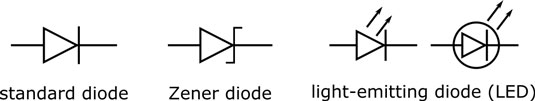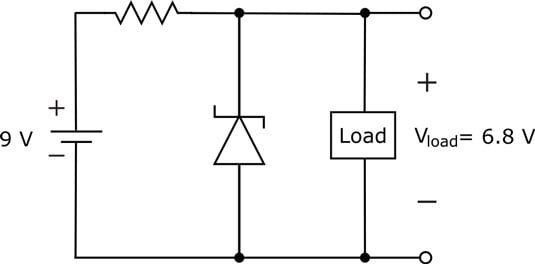##### Electronics For DummiesZener diodes are special diodes that are meant to break down. They are really just heavily doped diodes that break down at much lower voltages than standard diodes. When you reverse-bias a Zener diode, and the voltage across it reaches or exceeds its breakdown voltage, the Zener diode suddenly starts conducting current backward through the diode (from cathode to anode).

As you continue to increase the reverse-biased voltage beyond the breakdown point, the Zener continues to conduct more and more current — while maintaining a steady voltage drop.

Keep in mind these two important ratings for Zener diodes:

• The breakdown voltage, commonly called the Zener voltage, is the reverse-biased voltage that causes the diode to break down and conduct current. Breakdown voltages, which are controlled by the semiconductor doping process, range from 2.4 V to hundreds of volts.

• The power rating tells you the maximum power (voltage x current) the Zener diode can handle. (Even diodes designed to break down can really break down if you exceed their power ratings.)

To see the circuit symbol for a Zener diode, check out this image.Symbols used to indicate diodes.

Because Zener diodes are so good at maintaining a constant reverse-biased voltage, even as current varies, they're used to regulate voltage in circuits. In the circuit shown, for example, a 9 V DC supply is being used to power a load, and a Zener diode is placed so that the DC supply exceeds the breakdown voltage of 6.8 V. (Note that this voltage is reverse-biasing the diode.) Because the load is in parallel with the Zener diode, the voltage drop across the load is the same as the Zener voltage, which is 6.8 V. The remaining supply voltage is dropped across the resistor (which is there to limit the current through the diode so the power rating is not exceeded).The Zener diode stabilizes the voltage drop across the load in this circuit.

Here's the important thing: If the supply voltage varies up or down around its nominal 9 V value, the current in the circuit will fluctuate but the voltage across the load will remain the same: a constant 6.8 V. The Zener diode allows current fluctuations while stabilizing the voltage, whereas the resistor voltage varies as the current fluctuates.